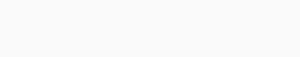#Quants Questions : Pipes & Cistern Set 1

Hello Aspirants. Welcome to Online Quantitative Aptitude Section with explanation in AffairsCloud.com. Here we are creating question sample in Pipes & Cistern, which is common for all competitive exams. We have included Some questions that are repeatedly asked in bank exams !!!

1. Two pipes can fill a tank in 6hours and 8 hours .While a third pipe empties the full tank in 12hours.If all the three pipes are operate simultaneously, In how much time  will the tank be filled ?
A)4 hours
B)4hours 48min
C)5hours
D)5hours 48min
E)None of these
Explanation :
1/6+1/8 – 1/12 =(4+3-2)/24 = 5/24
Time taken to fill the tank = 24/5 = 4hours 48min

2. A tap can fill a tank in 6hrs.After half the tank is filled, three more similar taps are opened. What is the total time taken to fill the tank completely ?
A)3hrs
B)3hrs 15 min
C)3hrs 45 min
D)4hrs
E)None of these
Explanation :
Time taken to fill the half tank = 6/2 = 3 hrs.—(1/2 part is filled)
Remaining part = ½
Time taken to fill the remaining part = [1/(4×1/6)] ×(1/2) = (3×1)/(2×2) = 3/4hrs
¾ hrs = 45 min
Total time = 3hrs+45 min = 3hrs 45min

3. Two pipes can fill a tank in 8 hours and 10 hours .If two pipes are operate simultaneously, In how much time  will the tank be filled ?
A)4hrs 18min
B)10min
C)4hrs
D)12hrs 10min
E)None of these
Explanation :
1/(A+B) =1/8 + 1/10 = (10+8)/80 = 18/80 = 9/40
40/9=4hrs 18min to filled the tank

4. Two pipes  A and B  can fill a tank in 20 minutes and 40 minutes.If both pipes are opened simultaneously , after how much time should B be closed  so that the tank is full in 18 minutes ?
A)10 min
B)9 min
C)8 min
D)7min
E)None of these
Answer -5) None of these (4)
Explanation :
A fill the tank in 1 minute (20×2 = 40) = 2 units
B fill the tank in 1 minute (40×1 = 40) =  1units
For 18 min(A) = 18×2 = 36 units
Remaining = 40 – 36 = 4 units
Time for B be closed  so that the tank is full in 18 minutes = 4/1 = 4 min

5. A pump can fill the tank in  4 hours.Because of a leak in the tank i took 5(1/2) hours to fill the tank.If the tank is full,how much time will the leak take to empty it ?
A)14hrs
B)14hrs 20min
C)14hrs 40min
D)14hrs 45min
E)None of these
Explanation:6. Three taps  P,Q and R can fill a tank in 10,20 and 30 hours respectively. If A is open all the time and Q and R are open for one hour each alternatively ,the tank will be fill in
A)7 hours
B)7 hours 30min
C)7hours 45min
D)7hours 20 min
E)None of these
Explanation :
A+B in 1 hr =(1/10)+(1/20) = (2+1)/20 =  3/20
A+C in 1 hr =(1/10)+(1/30) = (3+1)/20 =  4/30
In 2 hr => (3/20)+(4/30) = (9+8)/60 = 17/60
Part filled in 6 hours =3×(17/60) = 17/20
Remaining part = 1-(17/20) =(20 – 17)/20= 3/20(filled by A and B)……1hour
Total time taken to fill the tank = 6+1 = 7 hours

7. One pipe can fill a tank twice as fast as another pipe.If together  the two pipes can fill the tank in 12 minutes, then the slower pipe alone will be able to fill the tank in
A)30min
B)33min
C)36min
D)35min
E)None of these
Explanation :
(1/x)+(1/2x)=(1/12)
(3/x)= (1/12)=> (x/3) = 12
X = 36 min

8. 10 buckets of water fill a tank , when the capacity of each bucket is 11.5 litres.How many buckets  will be needed to fill the same tank, If the capacity of each bucket is 5 litres ?
A)22
B)32
C)21
D)23
E)None of these
Explanation :
Capacity of the tank = 10×11.5 = 115
Capacity of bucket = 5 litres
Number of buckets needed = 115/5 =23

9. Two pipes fill the tank in 12min and 15min.There is also a waste pipe in the tank.When all the three are opened,the empty tank is full in 20 min.How long will the waste pipe take to empty the tank ?
A)10 min
B)20 min
C)30 min
D)35 min
E)None of these
Explanation :
(1/20) –((1/12)+(1/15)) = (1/20) – [(5+4)/60]
= (3-9)/60 = -6/60
= -1/10= 10 min

10. Two pipes can fill a tank in 4 hours and 12 hours .If two pipes are operate simultaneously, In how much time  will the tank be filled ?
A)3hrs
B)3 hrs 10min
C)13hrs
D)3hrs 10min
E)None of these